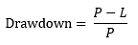# Forex Drawdown Calculator

Aug 10, 2023Forex Calculator

 Starting Balance: Consecutive Losses (Periods): Loss per Trade (%):

Ending Balance:

Total Loss (%):

Periods Starting Balance Ending Balance Total Loss Total Loss (%)

Forex Drawdown calculator is a Forex calculator that gives you ending balance and total loss on your trading account after a number of consecutive losses.

The drawdown calculator requires three variables to be entered and you get a table with the results.

You can use this calculator in trading to see what you could experience if you have a number of bad trades in a row. This information is useful for a trader to see how much capital you will have after a losing streak happens.

## What is Drawdown in Forex

Drawdown in Forex is the amount reduced in the account balance caused by the consecutive losses. And drawdown is calculated like an account balance difference from account balance peak to account balance low caused by that consecutive losses.

For example if you have \$10 000 at the start and you have 2 bad trades in a row. Each trade has 2% risk which means you will lose 2 x 1% = 2% (roughly calculated).

\$10 000 – \$200 = \$9 800

So the account balance peak was \$10 000 and you have ended at \$9 800 which is account low.

The drawdown on this account is \$200 from \$10 000 to \$9 000.

But this is a quick overview of how drawdown works, but there is a formula you can use to get the calculation correct.

## What is the Formula for Drawdown

Formula for drawdown is equal to:P = Peak Balance

L = Lowest Balance (valley)

### How Do You Calculate Drawdown in Forex?

In the case from above where the account started from \$10 000 and ended on \$9 800 here is drawdown calculated.

Drawdown = (10 000 – 9 900) / 10 000 = 100 / 10 000 = 0.01

Drawdown in % = 0.01 x 100 = 1%

Now, on the second trade you start with another account balance and that is \$10 000 – \$100 = \$9 900. Now you have another bad trade with 1% risk.

1% risk on \$9 900 = \$99.

Drawdown = (9 900 – 9 801) / 9 900 = 99 / 9 900 = 0.01

Drawdown in % = 0.01 x 100 = 1%

Now you have ended up with \$9 801 after 2 bad trades which is difference of 1.99%:

(\$9 801 – \$10 000) / \$ 10 000 = 0.0199

in % = 0.0199 x 100 = 1.99%

## What is a Drawdown Calculator?

Drawdown calculator in Forex is a tool that calculates account balance drop from peak to valley caused by the consecutive losses.

You can enter starting balance, number of traders and the risk per each trade and as a result you get ending balance with percentage drop.

You also get a table with the results so you can see how the results change on each trade you lose.

### How to Use the Drawdown Calculator

If you put the numbers in the drawdown calculator from the example I have shown you where drawdown is 1.99% you would get this.You can see in the table below where the drawdown calculator gives you each step in calculation.

You can see that the calculator quickly calculates all the trades for you where you define starting balance, number of trades and the risk per each trade.

You get an ending balance and total loss in percentage after drawdown is calculated.

## Conclusion

Drawdown calculator in Forex is a useful tool that shows you what you would end up with after consecutive losses on your trading account.

The drawdown calculator requires you to have constant risk per each trade. If you would like to change the risk per trade you would need to manually calculate each of the steps.

Neither GETKNOWTRADING.com nor its employee or affiliates will be held responsible for the reliability or accuracy of this data. The service is provided in good faith.

However, there are no explicit or implicit warranties of accuracy. The user agrees not to hold GETKNOWTRADING.com or any of its affiliates, liable for trading decisions that are based on the calculators from this website.

“Disclosure: Some of the links in this post are “affiliate links.” This means if you click on the link and purchase the item, I will receive an affiliate commission. This does not cost you anything extra on the usual cost of the product, and may sometimes cost less as I have some affiliate discounts in place I can offer you”#### Frano Grgić

A Forex trader since 2009. I like to share my knowledge and I like to analyze the markets. My goal is to have a website which will be the first choice for traders and beginners. Market analysis is featured by Forex Factory next to large publications like DailyFX, Bloomberg... GetKnowTrading is becoming recognized among traders as a website with simple and effective market analysis.

##### Forex Calculator

A list of all Forex Calculators